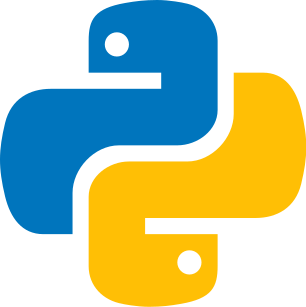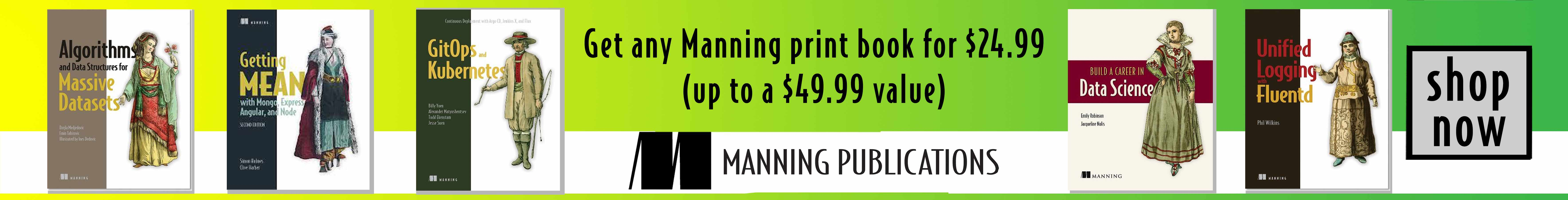Last update: February 11th, 2022

A set is a collection of data. Thus, the set of integers between 1 and 3 is written: { 1; 2; 3 }. We are going to see with Python how to implement them.### Definition of a set

We will study the different characteristics using Python's set.

Here we represent the sets with objects in crates. So there is a set of fruits, a set of pens and a set of books.

#### Codepython
1set([0, 1, 2, 3])

You can add one element using add() and several elements using update().

Here we want to add one or more fruits to those already in the crate. So one fruit is added to the second comic strip, and two fruits are added to the third comic strip.

#### Codepython
1s = set([1, 33, 67])
3
4print(s) # {1, 2, 67, 33}
5
6# Update
7s.update([26, 12, 9, 14])
8
9# Copy
10s2 = s.copy()
11print(s2) # {1, 2, 67, 33, 9, 12, 14, 26}

### Remove an item

You can remove a single element using .pop() (start of the set) or remove a specific element using .remove().

Here we want to remove the fruit. In the second comic board, the fruit placed in the first position is removed automatically, and in the last comic board, it is the one we have designated that is removed.

#### Codepython
1s = set([1, 2, 3, 4, 5, 6])
2s.pop()
3
4print(s) # {2, 3, 4, 5, 6}
5
6set([2,3,4,5,6])
7
8s.remove(3)
9
10print(s)  # {2, 4, 5, 6}

### Copy a set

You can copy a set with .copy().

Here is a simple copy of the fruit crate in the second comic strip and the last comic strip a copy of the book crate.

#### Codepython
1s = set([1, 33, 67])
2s2 = s.copy()
3print(s2) # {1, 67, 33}

### Union

This is the set of elements that are in A OR in B. It is denoted by A ∪ B.

The union is represented here with two crates placed side by side with their contents, the fruits, and the yellow books that form a new set.

#### Codepython
1s1 = set([4, 6, 9])
2s2 = set([1, 6, 8])
3s3 = s1.union(s2)
4print(s3)  # {1, 4, 6, 8, 9}
5

### Intersection

The intersection of A and B is a new set that includes all elements that belong to both A and, at the same time to B. It is denoted A B ∩, i.e., A AND B. We will therefore recover the common numbers between two sets.

In these comic strips, the intersection is explained by the yellow books on the side of each crate. So, initially, there were two crates that were assembled. The contents of these two crates were mixed, and in the end, they have elements in common, the yellow books, surrounded by a black rectangle. This is the intersection.

#### Codepython
1s1 = set([4, 6, 9])
2s2 = set([1, 6, 8])
3s3 = s1.intersection(s2)
4print(s3)  # {6}
5

### Difference

We are going to recover the member elements of a first set not present in the second and not common.

To illustrate the difference, we put the crate's contents in the first comic strip in two crates side by side. We will then compare the contents of the first crate to the second and select the fruits that are not in common with a black rectangle.

#### Codepython
1s1 = set([4, 6, 9])
2s2 = set([1, 6, 8])
3s3 = s1.difference(s2)
4print(s3)  # {9, 4}
5

### Symmetrical difference

Here we recover all the differences of the two sets except the common points (i.e. the intersection).

Here we will divide the crate contents and select all the non-common elements with a black rectangle. We see the only common element, which is the banana.

#### Codepython
1s1 = set([4, 6, 9])
2s2 = set([1, 6, 8])
3s3 = s1.symmetric_difference(s2)
4print(s3)  # {1, 4, 8, 9}
5A set is a collection of data. We can perform several operators (addition, deletion) in order to manipulate them. We can use the Set of Python.